# xTRMM (Triangle Matrix-Matrix Product) VI

LabVIEW 2012 GPU Analysis Toolkit Help

Edition Date: June 2012

Part Number: 373575A-01

»View Product InfoDownload Help (Windows Only)

Owning Palette: LVCUBLAS

Requires: GPU Analysis Toolkit

Calculates the triangular matrix expression of the form alpha*op(A)*B or alpha*B*op(A) for triangular matrix A, for rectangular matrices B and C (if applicable), for scalars alpha and beta, and for matrix operation op(X). When you wire data to A in, B in (if available), and C, this VI automatically selects the first available instance.

To use a different instance, you must manually select the polymorphic instance you want to use.Note  The expressions that incorporate use of a matrix C to store the results offer better performance than those that operate on matrix B inplace.

Use the pull-down menu to select an instance of this VI.

 Select an instance xTRMM (Triangle Matrix-Matrix Product) (aAB)xTRMM (Triangle Matrix-Matrix Product) (aBA)xTRMM (Triangle Matrix-Matrix Product) (C=aAB)xTRMM (Triangle Matrix-Matrix Product) (C=aBA)

## xTRMM (Triangle Matrix-Matrix Product) (aAB)

The connector pane displays the default data types for this polymorphic instance.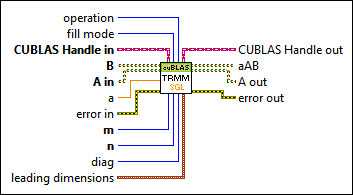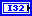operation specifies the operation the VI performs on matrix A, which results in matrix op(A) that can equal A, A' or conj(A')'.

 0 CUBLAS_OP_N (default)—Specifies A. 1 CUBLAS_OP_T—Specifies A'. 2 CUBLAS_OP_C—Specifies conj(A')'.fill mode specifies the triangular portion of the matrix A in the calculation.

 0 CUBLAS_FILL_MODE_LOWER (default) 1 CUBLAS_FILL_MODE_UPPERCUBLAS Handle in specifies the initialized CUBLAS library to use for the BLAS calculation. For example, you can wire the CUBLAS Handle output from the Initialize Library VI to specify the CUBLAS handle to the CUBLAS library you already initialized. This input also determines the device that executes the function.B specifies the matrix B stored on the device. This input specifies a class that can contain the following data types:

• Single-precision, floating-point numeric
• Double-precision, floating-point numeric
• Complex single-precision, floating-point numeric
• Complex double-precision, floating-point numericA in specifies the triangular matrix A stored on the device. This input specifies a class that can contain the following data types:

• Single-precision, floating-point numeric
• Double-precision, floating-point numeric
• Complex single-precision, floating-point numeric
• Complex double-precision, floating-point numeric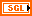a represents alpha and specifies the scalar operand in the product alpha*op(A)*B. The default is 1.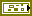error in describes error conditions that occur before this node runs. This input provides standard error in functionality.m specifies the number of rows to use in matrix op(A) and B.n specifies the number of columns to use in B.diag specifies the value of the diagonal elements of the triangular matrix A.

 0 CUBLAS_DIAG_NON_UNIT (default) 1 CUBLAS_DIAG_UNIT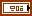leading dimensions specifies the column dimension to index consecutive rows. Use lda, ldb, and ldc for A, B, and C, respectively.CUBLAS Handle out returns the handle that defines the BLAS operation.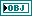aAB returns a matrix with the same dimensions as B. For the elements of the first m rows and n columns of the triangular component defined by fill mode, the VI returns the result of alpha*op(A)*B. For any remaining elements, the VI returns the value of the element with the same index in B.A out returns the triangular matrix A stored on the device.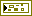error out contains error information. This output provides standard error out functionality.

## xTRMM (Triangle Matrix-Matrix Product) (aBA)

The connector pane displays the default data types for this polymorphic instance.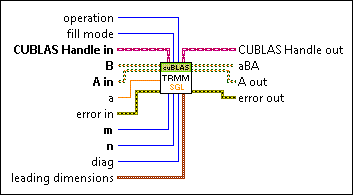operation specifies the operation the VI performs on matrix A, which results in matrix op(A) that can equal A, A' or conj(A')'.

 0 CUBLAS_OP_N (default)—Specifies A. 1 CUBLAS_OP_T—Specifies A'. 2 CUBLAS_OP_C—Specifies conj(A')'.fill mode specifies the triangular portion of the matrix A in the calculation.

 0 CUBLAS_FILL_MODE_LOWER (default) 1 CUBLAS_FILL_MODE_UPPERCUBLAS Handle in specifies the initialized CUBLAS library to use for the BLAS calculation. For example, you can wire the CUBLAS Handle output from the Initialize Library VI to specify the CUBLAS handle to the CUBLAS library you already initialized. This input also determines the device that executes the function.B specifies the matrix B stored on the device. This input specifies a class that can contain the following data types:

• Single-precision, floating-point numeric
• Double-precision, floating-point numeric
• Complex single-precision, floating-point numeric
• Complex double-precision, floating-point numericA in specifies the triangular matrix A stored on the device. This input specifies a class that can contain the following data types:

• Single-precision, floating-point numeric
• Double-precision, floating-point numeric
• Complex single-precision, floating-point numeric
• Complex double-precision, floating-point numerica represents alpha and specifies the scalar operand in the product alpha*B*op(A). The default is 1.error in describes error conditions that occur before this node runs. This input provides standard error in functionality.m specifies the number of rows to use in B.n specifies the number of columns to use in matrix op(A) and B.diag specifies the value of the diagonal elements of the triangular matrix A.

 0 CUBLAS_DIAG_NON_UNIT (default) 1 CUBLAS_DIAG_UNITleading dimensions specifies the column dimension to index consecutive rows. Use lda, ldb, and ldc for A, B, and C, respectively.CUBLAS Handle out returns the handle that defines the BLAS operation.aBA returns a matrix with the same dimensions as B. For the elements of the first m rows and n columns of the triangular component defined by fill mode, the VI returns the result of alpha*B*op(A). For any remaining elements, the VI returns the value of the element with the same index in B.A out returns the triangular matrix A stored on the device.error out contains error information. This output provides standard error out functionality.

## xTRMM (Triangle Matrix-Matrix Product) (C=aAB)

The connector pane displays the default data types for this polymorphic instance.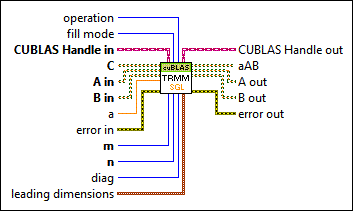operation specifies the operation the VI performs on matrix A, which results in matrix op(A) that can equal A, A' or conj(A')'.

 0 CUBLAS_OP_N (default)—Specifies A. 1 CUBLAS_OP_T—Specifies A'. 2 CUBLAS_OP_C—Specifies conj(A')'.fill mode specifies the triangular portion of the matrix A in the calculation.

 0 CUBLAS_FILL_MODE_LOWER (default) 1 CUBLAS_FILL_MODE_UPPERCUBLAS Handle in specifies the initialized CUBLAS library to use for the BLAS calculation. For example, you can wire the CUBLAS Handle output from the Initialize Library VI to specify the CUBLAS handle to the CUBLAS library you already initialized. This input also determines the device that executes the function.C specifies the matrix C stored on the device. This input specifies a class that can contain the following data types:

• Single-precision, floating-point numeric
• Double-precision, floating-point numeric
• Complex single-precision, floating-point numeric
• Complex double-precision, floating-point numericA in specifies the triangular matrix A stored on the device. This input specifies a class that can contain the following data types:

• Single-precision, floating-point numeric
• Double-precision, floating-point numeric
• Complex single-precision, floating-point numeric
• Complex double-precision, floating-point numericB in specifies the matrix B stored on the device. This input specifies a class that can contain the following data types:

• Single-precision, floating-point numeric
• Double-precision, floating-point numeric
• Complex single-precision, floating-point numeric
• Complex double-precision, floating-point numerica represents alpha and specifies the scalar operand in the product alpha*op(A)*B. The default is 1.error in describes error conditions that occur before this node runs. This input provides standard error in functionality.m specifies the number of rows to use in matrix op(A) and C.n specifies the number of columns to use in B in and C.diag specifies the value of the diagonal elements of the triangular matrix A.

 0 CUBLAS_DIAG_NON_UNIT (default) 1 CUBLAS_DIAG_UNITleading dimensions specifies the column dimension to index consecutive rows. Use lda, ldb, and ldc for A, B, and C, respectively.CUBLAS Handle out returns the handle that defines the BLAS operation.aAB returns a matrix with the same dimensions as C. For the elements of the first m rows and n columns of the triangular component defined by fill mode, the VI returns the result of alpha*op(A)*B. For any remaining elements, the VI returns the value of the element with the same index in C.A out returns the triangular matrix A stored on the device.B out returns the matrix B stored on the device.error out contains error information. This output provides standard error out functionality.

## xTRMM (Triangle Matrix-Matrix Product) (C=aBA)

The connector pane displays the default data types for this polymorphic instance.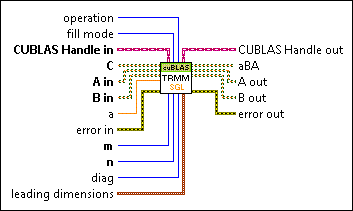operation specifies the operation the VI performs on matrix A, which results in matrix op(A) that can equal A, A' or conj(A')'.

 0 CUBLAS_OP_N (default)—Specifies A. 1 CUBLAS_OP_T—Specifies A'. 2 CUBLAS_OP_C—Specifies conj(A')'.fill mode specifies the triangular portion of the matrix A in the calculation.

 0 CUBLAS_FILL_MODE_LOWER (default) 1 CUBLAS_FILL_MODE_UPPERCUBLAS Handle in specifies the initialized CUBLAS library to use for the BLAS calculation. For example, you can wire the CUBLAS Handle output from the Initialize Library VI to specify the CUBLAS handle to the CUBLAS library you already initialized. This input also determines the device that executes the function.C specifies the matrix C stored on the device. This input specifies a class that can contain the following data types:

• Single-precision, floating-point numeric
• Double-precision, floating-point numeric
• Complex single-precision, floating-point numeric
• Complex double-precision, floating-point numericA in specifies the triangular matrix A stored on the device. This input specifies a class that can contain the following data types:

• Single-precision, floating-point numeric
• Double-precision, floating-point numeric
• Complex single-precision, floating-point numeric
• Complex double-precision, floating-point numericB in specifies the matrix B stored on the device. This input specifies a class that can contain the following data types:

• Single-precision, floating-point numeric
• Double-precision, floating-point numeric
• Complex single-precision, floating-point numeric
• Complex double-precision, floating-point numerica represents alpha and specifies the scalar operand in the product alpha*B*op(A). The default is 1.error in describes error conditions that occur before this node runs. This input provides standard error in functionality.m specifies the number of rows to use in B in and C.n specifies the number of columns to use in matrix op(A) and B.diag specifies the value of the diagonal elements of the triangular matrix A.

 0 CUBLAS_DIAG_NON_UNIT (default) 1 CUBLAS_DIAG_UNITleading dimensions specifies the column dimension to index consecutive rows. Use lda, ldb, and ldc for A, B, and C, respectively.CUBLAS Handle out returns the handle that defines the BLAS operation.aBA returns a matrix with the same dimensions as C. For the elements of the first m rows and n columns of the triangular component defined by fill mode, the VI returns the result of alpha*B*op(A). For any remaining elements, the VI returns the value of the element with the same index in C.A out returns the triangular matrix A stored on the device.B out returns the matrix B stored on the device.error out contains error information. This output provides standard error out functionality.

## xTRMM (Triangle Matrix-Matrix Product) Details

For more information on how to use this VI, refer to the Designing the Block Diagram to Compute on a GPU Device topic.

For more information about the CUBLAS library and BLAS operations, refer to the NVIDIA GPU Computing Documentation website at nvidia.com and download the CUBLAS Library User Guide.

Refer to the BLAS (Basic Linear Algebra Subprograms) website at netlib.org for more information on BLAS functions.